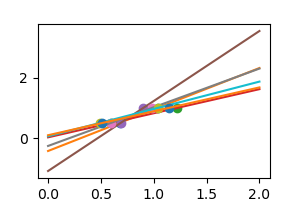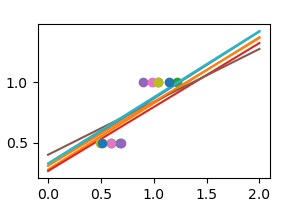# 3.6.10.7. Plot variance and regularization in linear models¶

```import numpy as np

# Smaller figures
from matplotlib import pyplot as plt
plt.rcParams['figure.figsize'] = (3, 2)
```

We consider the situation where we have only 2 data point

```X = np.c_[ .5, 1].T
y = [.5, 1]
X_test = np.c_[ 0, 2].T
```

Without noise, as linear regression fits the data perfectly

```from sklearn import linear_model
regr = linear_model.LinearRegression()
regr.fit(X, y)
plt.plot(X, y, 'o')
plt.plot(X_test, regr.predict(X_test))
```In real life situation, we have noise (e.g. measurement noise) in our data:

```np.random.seed(0)
for _ in range(6):
noisy_X = X + np.random.normal(loc=0, scale=.1, size=X.shape)
plt.plot(noisy_X, y, 'o')
regr.fit(noisy_X, y)
plt.plot(X_test, regr.predict(X_test))
```As we can see, our linear model captures and amplifies the noise in the data. It displays a lot of variance.

We can use another linear estimator that uses regularization, the `Ridge` estimator. This estimator regularizes the coefficients by shrinking them to zero, under the assumption that very high correlations are often spurious. The alpha parameter controls the amount of shrinkage used.

```regr = linear_model.Ridge(alpha=.1)
np.random.seed(0)
for _ in range(6):
noisy_X = X + np.random.normal(loc=0, scale=.1, size=X.shape)
plt.plot(noisy_X, y, 'o')
regr.fit(noisy_X, y)
plt.plot(X_test, regr.predict(X_test))

plt.show()
```Total running time of the script: ( 0 minutes 0.077 seconds)

Gallery generated by Sphinx-Gallery